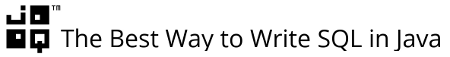{{announcement.body}}
{{announcement.title}}

# Ranking Functions: ROW_NUMBER(), RANK(), and DENSE_RANK()

DZone 's Guide to

# Ranking Functions: ROW_NUMBER(), RANK(), and DENSE_RANK()

### One of the most obvious and useful set of window functions are ranking functions where rows from your result set are ranked according to a certain scheme.

· Database Zone ·
Free Resource

Comment (0)

Save
{{ articles.views | formatCount}} Views

There was SQL before window functions and SQL after window functions

If you’re lucky enough to be using any of these databases, then you can use window functions yourself:

• CUBRID
• DB2
• Firebird
• Informix
• Oracle
• PostgreSQL
• SQL Server
• Sybase SQL Anywhere

(source here)

One of the most obvious and useful set of window functions are ranking functions where rows from your result set are ranked according to a certain scheme. There are three ranking functions:

• `ROW_NUMBER()`
• `RANK()`
• `DENSE_RANK()`

The difference is easy to remember. For the examples, let’s assume we have this table (using PostgreSQL syntax):

``````CREATE TABLE t(v) AS
SELECT * FROM (
VALUES('a'),('a'),('a'),('b'),
('c'),('c'),('d'),('e')
) t(v)``````

ROW_NUMBER()

… assigns unique numbers to each row within the `PARTITION` given the `ORDER BY` clause. So you’d get:

``````SELECT v, ROW_NUMBER() OVER()
FROM t``````

Note that some SQL dialects (e.g. SQL Server) require an explicit `ORDER BY` clause in the `OVER()` clause:

``````SELECT v, ROW_NUMBER() OVER(ORDER BY v)
FROM t
``````

The above query returns:RANK()

… behaves like `ROW_NUMBER()`, except that “equal” rows are ranked the same. If we substitute `RANK()` into our previous query:

``````SELECT v, RANK() OVER(ORDER BY v)
FROM t``````

… then the result we’re getting is this:As you can see, much like in a sports ranking, we have gaps between the different ranks. We can avoid those gaps by using

DENSE_RANK()

Trivially, `DENSE_RANK()` is a rank with no gaps, i.e. it is “dense”. We can write:

``````SELECT v, DENSE_RANK() OVER(ORDER BY v)
FROM t``````

… to obtainOne interesting aspect of `DENSE_RANK()` is the fact that it “behaves like” `ROW_NUMBER()` when we add the `DISTINCT` keyword.

``````SELECT DISTINCT v, DENSE_RANK() OVER(ORDER BY v)
FROM t``````

… to obtainIn fact, `ROW_NUMBER()` prevents you from using `DISTINCT`, because `ROW_NUMBER()` generates unique values across the partition before`DISTINCT` is applied:

``````SELECT DISTINCT v, ROW_NUMBER() OVER(ORDER BY v)
FROM t
ORDER BY 1, 2``````

`DISTINCT` has no effect:## Putting it all together

A good way to understand the three ranking functions is to see them all in action side-by-side. Run this query

``````SELECT
v,
ROW_NUMBER() OVER(ORDER BY v),
RANK()       OVER(ORDER BY v),
DENSE_RANK() OVER(ORDER BY v)
FROM t
ORDER BY 1, 2``````

… or this one (using the SQL standard `WINDOW` clause, to reuse window specifications):

``````SELECT
v,
ROW_NUMBER() OVER(w),
RANK()       OVER(w),
DENSE_RANK() OVER(w)
FROM t
WINDOW w AS (ORDER BY v)``````

… to obtain:Note that unfortunately, the `WINDOW` clause is not supported in all databases.

## SQL is awesome

These things can be written very easily using SQL window functions. Once you get a hang of the syntax, you won’t want to miss this killer feature in your every day SQL statements any more. Excited?Topics:
database, sql, window functions

Comment (0)

Save
{{ articles.views | formatCount}} Views

Published at DZone with permission of Lukas Eder , DZone MVB. See the original article here.

Opinions expressed by DZone contributors are their own.